# Community

Blog Events Webinars Tutorials Forum
×
Community Blog MaxCompute SQL Join Development Guide

# MaxCompute SQL Join Development Guide

In this article, we will be discussing several MaxCompute Join operations using standard SQL semantics.

By Sixiang

## Background

The results in this article are all derived using standard SQL semantics. We hope that you can write SQL statements according to the standard SQL semantics, to ensure the portability of subsequent SQL statements.

### JOIN ON Condition in MaxCompute SQL

One of the most common operations in MaxCompute SQL is Join. Currently MaxCompute provides several Join types: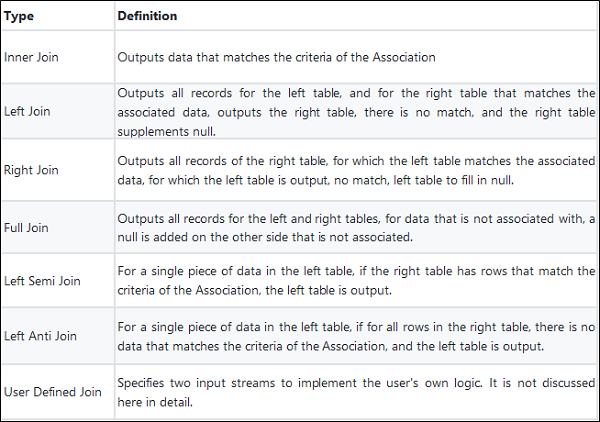Depending on the scenario, the user can use different Join types to implement the corresponding Association operation. But during actual implementation, users aren't always aware that the filtering criteria are different for JOIN ON and WHERE statements, or think they doing the same thing, for example, in a production environment, users can often be seen writing:

``````
A (LEFT/RIGHT/FULL/LEFT SEMI/LEFT ANTI) JOIN B

ON a.key = b.key and A.ds='20190121' and B.ds='20190121';
``````

The user intends to get the data for a partition in A and B for the Join operation, that is:

``````
(SELECT * FROM A WHERE ds='20190121') A

(LEFT/RIGHT/FULL/LEFT SEMI/LEFT ANTI)  JOIN

(SELECT * FROM B WHERE ds='20190121') B

ON a.key = b.key
``````

However, for different Join types, the two may not be equivalent. It cannot implement partition condition pushdown, causing both full table scanning and correctness issues. Here, we will briefly analyze the similarities and differences between the filter conditions.

1. WHERE condition of subquery
2. JOIN ON condition
3. WHERE condition after JOIN ON

## 1. Principle

First, we describe the computing order of JOIN and WHERE conditions, for the following code:

``````
(SELECT * FROM A WHERE {subquery_where_condition} A) A

JOIN

(SELECT * FROM B WHERE {subquery_where_condition} B) B

ON {on_condition}

WHERE {where_condition}
``````

the computing order is

1. {subquery_where_condition} in the subquery
2. {on_condition} of JOIN
3. The computing of the JOIN result set {where_condition}

For different Join types, filter clauses are placed in {subquery_where_condition},{on_condition}, and {where_condition}. Sometimes the results are consistent, and sometimes they are not. We discuss for different conditions:

## 2. Putting Everything to Practice

### 2.1 Preparation

First we create a table A:

``````
CREATE TABLE A AS SELECT * FROM VALUES (1, 20190121),(2, 20190121),(2, 20190122) t (key, ds);
``````
 key ds 1 20190121 2 20190121 2 20190122

Then their Cartesian product is: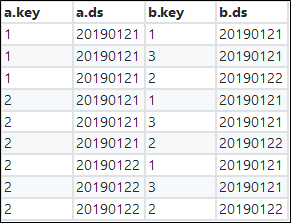### 2.2. Inner Join

Conclusion: The filter conditions in {subquery_where_condition}, {on_condition}, and {where_condition} are equivalent.

The processing logic of Inner Join is to create a Cartesian product with the left and right tables, then output rows that meet the ON expression.

The first case is to filter data by using a subquery:

``````
SELECT A.*, B.*

FROM

(SELECT * FROM A WHERE ds='20190121') A

JOIN

(SELECT * FROM B WHERE ds='20190121') B

ON a.key = b.key;
``````

There is only one result.

 a.key a.ds b.key b.ds 1 20190121 1 20190121

The second case is to filter data by using the JOIN condition:

``````
SELECT A.*, B.*

FROM A JOIN B

ON a.key = b.key and A.ds='20190121' and B.ds='20190121';
``````

There are 9 results, and only one of which meets the ON condition.

 a.key a.ds b.key b.ds 1 20190121 1 20190121

The third case is to filter data by using the WHERE condition after JOIN.

``````
SELECT A.*, B.*

FROM A JOIN B

ON a.key = b.key

WHERE A.ds='20190121' and B.ds='20190121';
``````

Of the 9 Cartesian product results, three results meet the ON condition "a.key = b.key":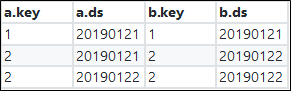Filter this result set by using the condition "A.ds='20190121' and B.ds='20190121'". Only one result is returned, which is consistent with the previous result.

 a.key a.ds b.key b.ds 1 20190121 1 20190121

### 2.3 Left Join

Conclusion: The filtering conditions in {subquery_where_condition}, {on_condition}, and {where_condition} are not necessarily equivalent.

For the filtering conditions for the left table, the ones placed in {subquery_where_condition} and {where_condition} are equivalent.

For the filter conditions for the right table, the ones placed in {subquery_where_condition} and {on_condition} are equivalent.

The processing logic of Left Join is to make the left and right tables a Cartesian product, then output rows that meet the ON expression. For the rows in the left table that do not meet the ON expression, the left table is output, and the NULL is returned in the right table.

The first case is to filter data by using a subquery:

``````
SELECT A.*, B.*

FROM

(SELECT * FROM A WHERE ds='20190121') A

LEFT JOIN

(SELECT * FROM B WHERE ds='20190121') B

ON a.key = b.key;

After filtering, there are two records on the left, one record on the right, and two records in the results.
``````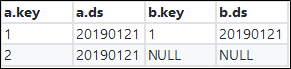The second case is to filter data by using the JOIN condition:

``````
SELECT A.*, B.*

FROM A LEFT JOIN B

ON a.key = b.key and A.ds='20190121' and B.ds='20190121';
``````

Of the nine Cartesian product results, also only one result meets the ON condition. The two remaining entries in the left table are returned and NULL are returned in the right table.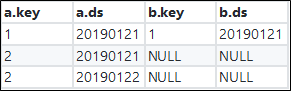The third case is to filter data by using the WHERE condition after JOIN.

``````
SELECT A.*, B.*

FROM A LEFT JOIN B

ON a.key = b.key

WHERE A.ds='20190121' and B.ds='20190121';
``````

Of the 9 Cartesian product results, three results meet the ON condition "a.key = b.key":Filter this result set by using the condition "A.ds='20190121' and B.ds='20190121'". Only one result is returned.

 a.key a.ds b.key b.ds 1 20190121 1 20190121

As you can see, three different results have been obtained by placing the filter conditions in three different places.

### 2.4 Right Join

The Right Join and Left Join are similar, with the difference being between the left and right tables.

Conclusion: The filtering conditions in {subquery_where_condition}, {on_condition}, and {where_condition} are not necessarily equivalent.

For the filter conditions for the right table, the ones placed in {subquery_where_condition} and {where_condition} are equivalent.

For the filter conditions for the left table, the ones placed in {subquery_where_condition} and {on_condition} are equivalent.

### 2.5 Full Join

Conclusion: Putting filter conditions in {subquery_where_condition}, {on_condition}, and {where_condition} are non-equivalent.

The processing logic of Full Join is to make the left and right tables a Cartesian product, then output rows that meet the ON expression. For the rows on both tables that do not meet the ON expression, the table with data is output, and NULL is returned in the other table.

The first case is to filter data by using a subquery:

``````
SELECT A.*, B.*

FROM

(SELECT * FROM A WHERE ds='20190121') A

FULL JOIN

(SELECT * FROM B WHERE ds='20190121') B

ON a.key = b.key;
``````

After filtering, there are two on the left and two on the right, and three in the results: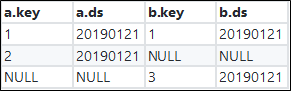The second case is to filter data by using the JOIN condition:

``````
SELECT A.*, B.*

FROM A FULL JOIN B

ON a.key = b.key and A.ds='20190121' and B.ds='20190121';
``````

Of the nine Cartesian product results, also only one result meets the ON condition. The two remaining entries in the left table are returned and NULL are returned in the right table. The two remaining entries in the right table are returned and NULL values are returned in the left table.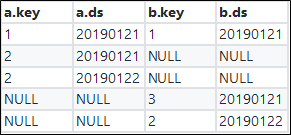The third case is to filter data by using the WHERE condition after JOIN.

``````
SELECT A.*, B.*

FROM A FULL JOIN B

ON a.key = b.key

WHERE A.ds='20190121' and B.ds='20190121';
``````

Of the nine Cartesian product results, three results meet the ON condition "a.key = b.key":Rows in one table that have no matches in the other table are returned, and NULL is put for the rows in the table where matches are not found to obtain a result set: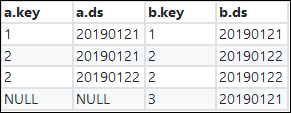Filter this result set by using the condition "A.ds='20190121' and B.ds='20190121'". Only one result is returned.

 a.key a.ds b.key b.ds 1 20190121 1 20190121

We can see that, similar to LEFT JOIN, three different results are obtained.

### 2.6 Left Semi Join

Conclusion: Putting a filtering condition in {subquery_where_condition}, {on_condition}, and {where_condition} are equivalent.

The processing logic of LEFT SEMI JOIN is that it tries to find a match in the right table for each entry in the left table and returns entries in the left table for which matching entries are found in the right table. Note that filtering conditions cannot be written in the WHERE condition for the right table after JOIN because LEFT SEMI JOIN only returns entries from the left table. LEFT SEMI JOIN is often used to implement the EXISTS semantics.

The first case is to filter data by using a subquery:

``````
SELECT A.*

FROM

(SELECT * FROM A WHERE ds='20190121') A

LEFT SEMI JOIN

(SELECT * FROM B WHERE ds='20190121') B

ON a.key = b.key;
``````

After filtering, two matching entries are found respectively in the right table and left table, but only one entry matches the "a.key = b.key" condition.

a.key a.ds
1 20190121

The second case is to filter data by using the JOIN condition:

``````
SELECT A.*

FROM A LEFT SEMI JOIN B

ON a.key = b.key and A.ds='20190121' and B.ds='20190121';
``````

Of the three records in the left table, also only one meets the ON condition.

a.key a.ds
1 20190121

The third case is to filter data by using the WHERE condition after JOIN.

``````
SELECT A.*

FROM A LEFT SEMI JOIN

(SELECT * FROM B WHERE ds='20190121') B

ON a.key = b.key

WHERE A.ds='20190121';
``````

Only one entry in the left table meets the ON condition.

a.key a.ds
1 20190121

Use "A.ds='20190121'" to filter the results. Again the result set only has one matching entry.

a.key a.ds
1 20190121

We can see that, like INNER JOIN, LEFT SEMI JOIN always obtains the same result, regardless of the position of the filtering condition.

### 2.7 Left Anti Join

Conclusion: Putting a filtering condition in {subquery_where_condition}, {on_condition}, and {where_condition} respectively are not necessarily equivalent.

For the filtering conditions for the left table, the ones placed in {subquery_where_condition} and {where_condition} are equivalent.

It is also equivalent to put the filtering condition for the right table in {subquery_where_condition} and {on_condition}. However, the expression for the right table cannot be put in {where_condition}.

The processing logic of LEFT ANTI JOIN is that it tries to find a match in the right table for each entry in the left table and returns entries in the left table if none of the entries in the right table are matched. Similarly, filtering conditions cannot be written in the Where condition for the right table after JOIN because LEFT ANTI JOIN only returns entries from the left table. LEFT SEMI JOIN is often used to implement the NOT EXISTS semantics.

The first case is to filter data by using a subquery:

``````
SELECT A.*

FROM

(SELECT * FROM A WHERE ds='20190121') A

LEFT ANTI JOIN

(SELECT * FROM B WHERE ds='20190121') B

ON a.key = b.key;
``````

After filtering, two entries are returned from the right table and two from the left table. The final result set only contains one entry.

 a.key a.ds 2 20190121

The second case is to filter data by using the JOIN condition:

``````
SELECT A.*

FROM A LEFT ANTI JOIN B

ON a.key = b.key and A.ds='20190121' and B.ds='20190121';
``````

Of the three entries from the left table, only one meets the ON condition, so the other two entries are returned.

 a.key a.ds 2 20190121 2 20190122

The third case is to filter data by using the WHERE condition after JOIN.

``````
SELECT A.*

FROM A LEFT ANTI JOIN

(SELECT * FROM B WHERE ds='20190121') B

ON a.key = b.key

WHERE A.ds='20190121';
``````

Two entries in the left table meet the ON condition.

 a.key a.ds 2 20190121 2 20190122

Then use "A.ds='20190121'" to filter the results. Only one entry meets the this condition.

 a.key a.ds 2 20190121

We can see that, for the LEFT ANTI JOIN operation, putting the same filtering condition in the JOIN ON condition and the WHERE condition respectively will return different results.

The preceding examples are only a test of writing filtering conditions in different places in the same application scenario and do not include the specific deduction process. This is even more complicated in scenarios where inequivalent expressions are involved. If you are interested, you can start deducing for yourself.

## 3. Summary

Putting the same filtering condition in different places may lead to drastically different semantics. If you want to perform JOIN operations only after data is filtered, simply remember the following considerations. If you still consider these rules too complex, the best way is to always write filtering conditions into subqueries, although this may look a little wordy.

1. For INNER JOIN/LEFT SEMI JOIN, you can write filtering expressions anywhere.
2. For LEFT JOIN/LEFT ANTI JOIN, you need to put a filtering condition for the left table in {subquery_where_condition} or {where_condition} and a filtering condition for the right table in {subquery_where_condition} or {on_condition}.
3. RIGHT JOIN is opposite to LEFT JOIN: You need to put a filtering condition for the right table in {subquery_where_condition} or {where_condition} and a filtering condition for the left table in {subquery_where_condition} or {on_condition}.
4. For FULL OUTER JOIN, filtering conditions can only be put in {subquery_where_condition}.
0 0 0
Share on

# Related Products

• ##Block Storage

Block-level data storage attached to ECS instances to achieve high performance, low latency, and high reliability

• ##Quick Starts

Deploy custom Alibaba Cloud solutions for business-critical scenarios with Quick Start templates.

• ##ApsaraDB for HBase

ApsaraDB for HBase is a NoSQL database engine that is highly optimized and 100% compatible with the community edition of HBase.

• ##Time Series Database (TSDB)

TSDB is a stable, reliable, and cost-effective online high-performance time series database service.